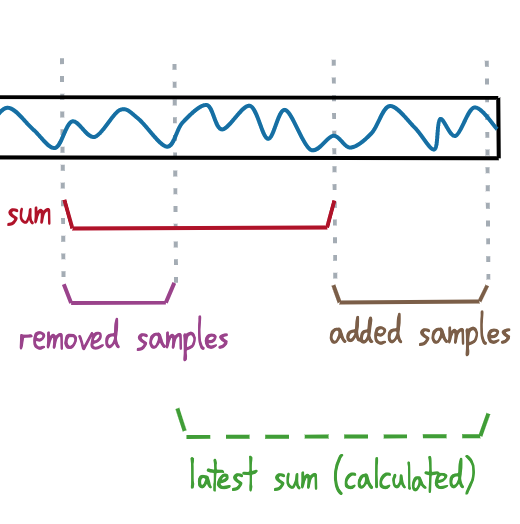# Box-filters without accumulating errors

Geraint Luff
Signalsmith Audio Ltd.

Exploring some ways to efficiently calculate box filters without accumulating floating-point errors, including varying-width filters.## The challenge

In audio/DSP, it's fairly common to need a rectangular moving-average (a.k.a "box filter"). This is the same as rolling sum of recent values divided by its own length:

This smooths out the signal a bit:A moving-average of 100 samples applied to an example input. The result is smoother, but also a bit delayed.

This is actually a form of lowpass, implemented by convolution. It has a finite-length impulse response, no overshoot, and can be computed efficiently (as seen below), which is a useful combination of properties in certain situations.

Here's the impulse-response of an un-normalised 100-sample box sum (i.e. box-filter without the 1/N factor):A 100-sample box filter applied to an impulse, making it obvious where the name comes from.

The most basic way to do it is direct convolution (i.e. adding together the most recent 100 samples) but that's slow - and gets worse for longer N.

## Better performance: cumulative sums

If you hold on to a previous result, you can calculate a new sum from it using only the samples that are added/removed:

If your moving-average window is progressing forward one sample at a time, maintaining the box-sum is very cheap:

If you're using fixed-point (integer) values, this works perfectly (as long as your `Sum` is a large enough type that nothing overflows).

But if you're using floating-point, additions and subtractions are slightly approximate - so your sum will drift over time.

## Avoiding floating-point errors

To avoid accumulating these floating-point errors, we occasionally reset our sum, to a more exact value which isn't based on our previous results (so it won't include previously-accumulated errors).

A straightforward approach is to start a separate "reset sum" from some convenient starting-point:

When our "reset" period and our "current" period match (N samples later) we replace our "running" sum with the "reset" sum.

As well as having to find your own `oldValue`, `resetStart()` and `resetEnd()` need to be called at specific times (N samples apart).

### Fixed-length period

If our box-sum has a fixed period, we can use a circular buffer that's exactly the length of our box-sum. This makes two things nice and simple:

• Our "new" value replaces our "old" value in the buffer (same index)
• We can perform a reset every N samples

If you already have a circular buffer of the right length, you can use the code above, calling `resetEnd()` and `resetStart()` whenever the index wraps around.

If not, here's some example code which includes a circular buffer:

### Variable-length box-sum

The previous approach works if your box-sum is always the same length - but if you want to stretch/shrink your box-sum period while it's running, you'll need something else.

In this case, we'll often have a write-head (inserting the latest values) and a read-head (returning delayed values) chasing each other around the circular buffer, and we're interested in the sum of the values which lie between them:

We start a new reset sum when the write-head wraps back around to 0:

When the read-head wraps around, our reset-sum will have the correct period to replace our running-sum:

If you're already handling your own circular buffer, you can just use the code above, calling `resetStart()` and `resetEnd()` as the appropriate heads wrap around.

#### Example code

It's a bit unwieldy, but here's some code for a variable-length box-sum with built-in buffer. It has `.push()` and `.pop()` methods (similar to a FIFO queue):

### Evan Balster's Bumpy Wheel

Evan Balster came up with an interesting solution to this, which he called the "Bumpy Wheel" (and is included in his rolling_stats C++ library).

Rather than maintain a value for the rectangular sum, this approach stores some "reference" sum, along with cumulative sums for the added/removed values:

The actual result is then calculated when needed:

When things progress far enough that entire reference period has been removed, we reset - using the "added" sum as our new "reference" sum, and resetting the added/removed sums to 0:The state of the Bumpy Wheel when it is ready for a reset - or a "bump" in Evan's code

Instead of being told when to do this, we can compare the "removed" and "reference" sums. Floating-point errors generally make comparing floating-point numbers slightly iffy, but because they are constructed from an identical sequence of additions, they will produce an identical result (including the exact same errors).

#### Comparison

The biggest advantage of this (compared to our previous variable-length approach) is that it can determine itself when to perform resets, without the user having to call `.resetStart()`/`.resetEnd()`.

Another advantage is that if you don't actually need the sum every time, you perform fewer calculations.

A slight disadvantage is that when you feed it a stream of 0s, it does a bit more calculation - but in my tests, the difference was pretty marginal (10%), and will depend on architecture/compiler/optimisation.

## Conclusion

But as ever: if you care about performance, trying a bunch of options and profiling is the only way to go. Evan himself ended up using a fixed-point version of a simple box-sum (which doesn't need resets with integers, but you do need know what range of values you put in, to protect against overflows) instead of the Bumpy Wheel, because he didn't need floating-point accuracy.

Anyway - I thought this was interesting, particularly the Bumpy Wheel stuff, and hopefully you did too. 😛